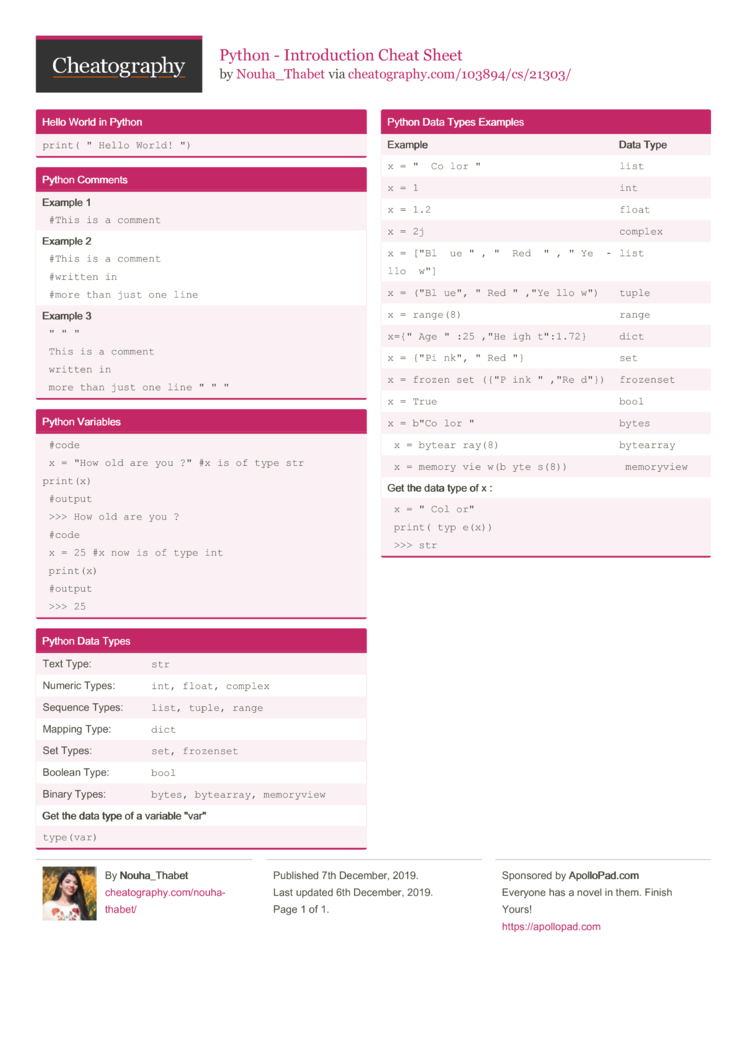# Python - Introduction Cheat Sheet by Nouha_Thabet

### Hello World in Python

 `print­("Hello World!­")`

 Example 1 ` #This is a comment ` Example 2 ` #This is a comment #written in #more than just one line ` Example 3 ` "­"­" This is a comment written in more than just one line "­"­" `

### Python Variables

 ` #code x = "How old are you ?" #x is of type str print(x) #output >>> How old are you ? #code x = 25 #x now is of type int print(x) #output >>> 25 `

### Python Data Types

 Text Type: `str` Numeric Types: `int, float, complex` Sequence Types: `list, tuple, range` Mapping Type: `dict` Set Types: `set, frozenset` Boolean Type: `bool` Binary Types: `bytes, bytearray, memory­view` Get the data type of a variable "­var­" `type(­var)`

### Python Data Types Examples

 Exam­ple Data Type `x = "­­Co­lor­"` `list` `x = 1` `int` `x = 1.2` `float` `x = 2j` `complex` `x = ["Bl­­ue­"­,­"­­Red­­"­,­"­Ye­­llo­­w"]` `list` `x = ("Bl­ue",­"­Red­"­,"Ye­llo­w")` `tuple` `x = range(8)` `range` `x={"Ag­e":2­5,"H­eig­ht":­1.72}` `dict` `x = {"Pi­nk",­"­Red­"}` `set` `x = frozen­set­({"P­ink­"­,"Re­d"})` `froze­nset` `x = True` `bool` `x = b"Co­lor­"` `bytes` ` x = bytear­ray(8) ` `bytea­rray` ` x = memory­vie­w(b­yte­s(8)) ` ` memory­view` Get the data type of x : ` x = "­Col­or" print(­typ­e(x)) >>> str `1 Page
//media.cheatography.com/storage/thumb/nouha-thabet_python-introduction.750.jpg

PDF (recommended)